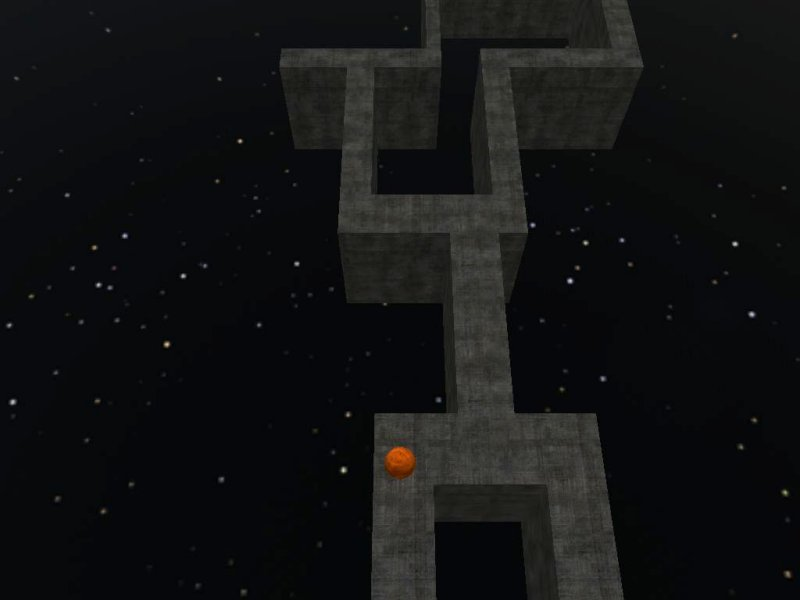Roller
 If you don't live on the moon, you have heard of Conitec's brand new lite-C, a new ide which incorporates the Acknex engine and allows us to create regular applications and small, 2D and 3D games easily. To prove that, I have improved the code from my lite-C physics workshop, creating a full game that looks like this:and uses less than 50 lines of code, just like this:   #include #include   VECTOR ball_speed; var soundtrack_handle; ENTITY* ball; SOUND* ball_wav = "ball.wav";   void kick_ball(void) // makes the ball jump when the player presses the "space" key {        VECTOR kick_speed;        kick_speed.x = 10;        kick_speed.y = 50 * (key_cul - key_cur);        kick_speed.z = 250; // ball jump height        vec_rotate(kick_speed, camera.pan);        phent_addvelcentral(ball, kick_speed);        snd_play (ball_wav, 100, 0); }   function main() {        video_screen = 1; // run in full screen mode        video_mode = 8; // set the resolution to 1024x768 pixels        on_space = kick_ball;        media_loop ("brittle.mp3", NULL, 50);        fps_max = 70;        level_load("roller.wmb"); // load the level        wait (2); // wait until the level is loaded        ent_createlayer("stars+6.tga", SKY | CUBE | VISIBLE, 0); // create the sky layer        ball = ent_create ("ball.mdl", vector(-38200, -30, 200), NULL); // create the ball        ph_setgravity (vector(0, 0, -386)); // set the gravity        phent_settype (ball, PH_RIGID, PH_SPHERE); // set the physics entity type        phent_setmass (ball, 3, PH_SPHERE); // and its mass        phent_setfriction (ball, 80); // set the friction        phent_setdamping (ball, 40, 40); // set the damping        phent_setelasticity (ball, 50, 20); // set the elasticity        while (ball.z > -1000)        {                ball_speed.x = 15 * (key_cur - key_cul); // move the ball using the cursor keys                ball_speed.y = 2.3 + 15 * (key_cuu - 0.15 * key_cud); // 25 sets the x / y movement speeds                ball_speed.z = 0; // no need to move on the vertical axis                phent_addtorqueglobal (ball, ball_speed); // add a torque (an angular force) to the ball                camera.x = ball.x - 300; // keep the camera 300 quants behind the ball                camera.y = ball.y; // using the same y with the ball                camera.z = ball.z + 1000; // and place it 1000 quants above the ball                camera.tilt = -60; // make it look downwards                wait (1);        }        while (key_any) {wait (1);} // wait until the player releases all the keys        media_stop (soundtrack_handle); // stop the music; another instance of it will run again shortly        main(); // and then run the game again }   Talk about productivity! The game uses a physics-based ball which can be maneuvered using the arrow keys; use the space key if the ball falls off a platform, but don't expect miracles - you won't be able to save it every time.   So what are you waiting for? Go here and get lite-C: http://www.conitec.net/english/gstudio/litec1.htm and install it, and then open the roller.c script from inside the \roller folder that is zipped inside Aum62's "Resources". Run the script and prepare for countless minutes of fun! (or hair pulling)